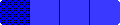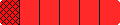Home

Grades 3-4 Fraction Bars Teacher's Guide

Also See: Standards for Grades 5 to 8
 NCTM STANDARDS 2000 ON TEACHING FRACTIONSEach of the following files has a statement from NCTM's Standards, and a corresponding page from the Grades 3-4 Teacher's Guide that illustrates the NCTM statement. Basic Concepts - part-to-whole models for fractions Equality - comparing shaded amounts Inequality - comparing fractions to 1/2 Fractions-Decimals-Percents - multiply symbols Addition - models for adding fractions Subtraction - models for subtracting fractions Multiplication - fractions times fractions

width="100%"width="100%"